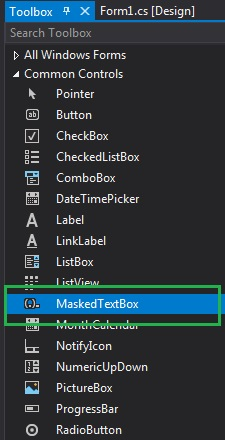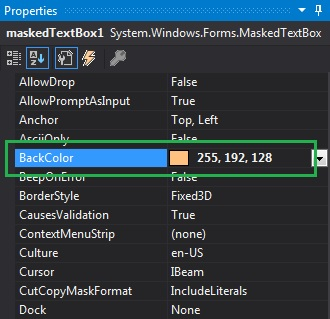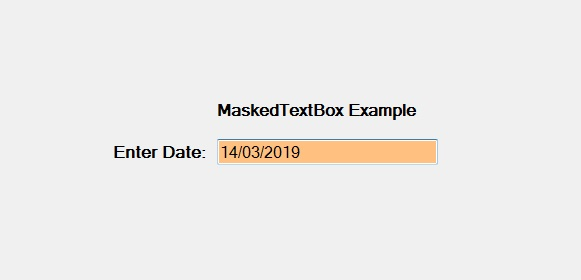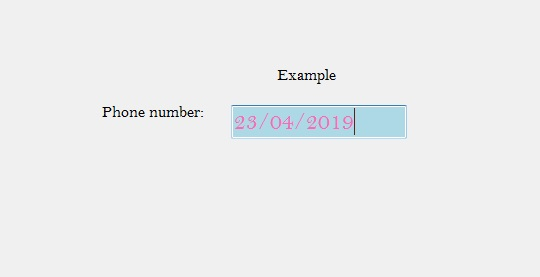Related Articles

# How to set the Background Color of the MaskedTextBox in C#?

• Last Updated : 26 Jul, 2019

In C#, MaskedTextBox control gives a validation procedure for the user input on the form like date, phone numbers, etc. Or in other words, it is used to provide a mask which differentiates between proper and improper user input. In MaskedTextBox control, you can set the background color of the MaskedTextBox using BackColor Property, which helps you to make your MaskedTextBox attractive. You can set this property in two different ways:

1. Design-Time: It is the easiest way to set the background color of the MaskedTextBox as shown in the following steps:

• Step 1: Create a windows form as shown in the below image:
Visual Studio -> File -> New -> Project -> WindowsFormApp• Step 2: Next, drag and drop the MaskedTextBox control from the toolbox on the form as shown in the below image:• Step 3: After drag and drop you will go to the properties of the MaskedTextBox and set the background color of the MaskedTextBox as shown in the below image:Output:2. Run-Time: It is a little bit trickier than the above method. In this method, you can set the background color of the MaskedTextBox control programmatically with the help of given syntax:

`public override System.Drawing.Color BackColor { get; set; }`

Here, Color indicates the background color of the MaskedTextBox control. The following steps show how to set the background color of the MaskedTextBox dynamically:

```// Creating a MaskedTextBox
```
• Step 2: After creating MaskedTextBox, set the BackColor property of the MaskedTextBox provided by the MaskedTextBox class.
```// Setting the Background color
m.BackColor = Color.LightBlue;
```
• Step 3: And last add this MaskedTextBox control to the form using the following statement:
```// Adding MaskedTextBox control on the form
 `using` `System;``using` `System.Collections.Generic;``using` `System.ComponentModel;``using` `System.Data;``using` `System.Drawing;``using` `System.Linq;``using` `System.Text;``using` `System.Threading.Tasks;``using` `System.Windows.Forms;`` ` `namespace` `WindowsFormsApp38 {`` ` `public` `partial` `class` `Form1 : Form {`` ` `    ``public` `Form1()``    ``{``        ``InitializeComponent();``    ``}`` ` `    ``private` `void` `Form1_Load(``object` `sender, EventArgs e)``    ``{``        ``// Creating and setting the ``        ``// properties of the Label``        ``Label l1 = ``new` `Label();``        ``l1.Location = ``new` `Point(413, 98);``        ``l1.Size = ``new` `Size(176, 20);``        ``l1.Text = ``" Example"``;``        ``l1.Font = ``new` `Font(``"Bell MT"``, 12);`` ` `        ``// Adding label on the form``        ``this``.Controls.Add(l1);`` ` `        ``// Creating and setting the ``        ``// properties of the Label``        ``Label l2 = ``new` `Label();``        ``l2.Location = ``new` `Point(242, 135);``        ``l2.Size = ``new` `Size(126, 20);``        ``l2.Text = ``"Phone number:"``;``        ``l2.Font = ``new` `Font(``"Bell MT"``, 12);`` ` `        ``// Adding label on the form``        ``this``.Controls.Add(l2);`` ` `        ``// Creating and setting the ``        ``// properties of MaskedTextBox``        ``MaskedTextBox m = ``new` `MaskedTextBox();``        ``m.Location = ``new` `Point(374, 137);``        ``m.Mask = ``"00/00/0000"``;``        ``m.Size = ``new` `Size(176, 20);``        ``m.Name = ``"MyBox"``;``        ``m.BorderStyle = BorderStyle.Fixed3D;``        ``m.BackColor = Color.LightBlue;``        ``m.ForeColor = Color.HotPink;``        ``m.Font = ``new` `Font(``"Bell MT"``, 18);`` ` `        ``// Adding MaskedTextBox ``        ``// control on the form``        ``this``.Controls.Add(m);``    ``}``}``}`